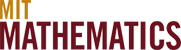# Computational Science & Numerical Analysis

Computational science is a key area related to physical mathematics. The problems of interest in physical mathematics often require computations for their resolution. Conversely, the development of efficient computational algorithms often requires an understanding of the basic properties of the solutions to the equations to be solved numerically. For example, the development of methods for the solution of hyperbolic equations (e.g. shock capturing methods in, say, gas-dynamics) has been characterized by a very close interaction between theoretical, computational, experimental scientists, and engineers.

Laurent Demanet

Alan Edelman

Steven Johnson

Gil Strang